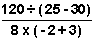# Practice Exercises for Pre-Algebra

Short Answer Directions: Read each question. Click once in an ANSWER BOX and type in your answer. After you click ENTER, a message will appear in the RESULTS BOX to indicate whether your answer is correct or incorrect. To start over, click CLEAR. Answers should be entered as integers.

Multiple Choice Directions: Select your answer by clicking on its button. Feedback to your answer is provided in the RESULTS BOX. If you make a mistake, choose a different button.

 1 Evaluate this arithmetic expression: 7 x 8 - 6 ÷ 2 + 18 x 3 ANSWER BOX:   RESULTS BOX:
 2 Evaluate this arithmetic expression:ANSWER BOX:   RESULTS BOX:
 3 Evaluate this arithmetic expression: 15 - 2 x 32 ÷ 6 ANSWER BOX:   RESULTS BOX:
 4 Evaluate this arithmetic expression: 36 ÷ (7-4)2 + 21 ANSWER BOX:   RESULTS BOX:
 5 Evaluate this arithmetic expression: [54 - 3(2 + 4)] ÷ -9 ANSWER BOX:   RESULTS BOX:
 6 Evaluate this arithmetic expression:ANSWER BOX:   RESULTS BOX:
 7. Which algebraic expression correctly represents this phrase? Twenty-five less than three times a number. 25 - 3x3 - 25x3x - 25None of the above. RESULTS BOX:
8.  Which algebraic expression correctly represents this phrase?
The quotient of a number and seven, decreased by ten.

 7p - 10 10 - 7pNone of the above.
 RESULTS BOX:
 9. Which sentence correctly represents this algebraic equation? 2w - 9 = 32 Nine less than twice a number is thirty-two.Nine is thirty-two more than twice a number.Nine is thirty-two less than twice a number.None of the above. RESULTS BOX:
 10. Which sentence correctly represents this algebraic equation?The quotient of a number and forty-two is seven.The quotient of forty-two and a number is seven.The quotient of seven and forty-two is a number.None of the above. RESULTS BOX: